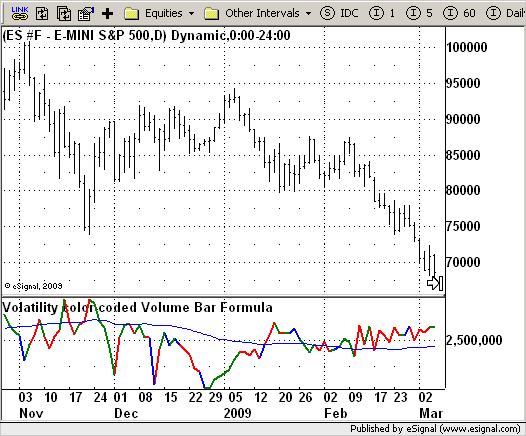# FVE Volatility color-coded Volume bar

ICE Data Services -

VolatilityVolumeBarFormula.efs
EFSLibrary - Discussion Board

File Name: VolatilityVolumeBarFormula.efs

Description:
FVE Volatility color-coded Volume bar

Formula Parameters:

• Samples : 22
• AvgLength : 50
• Cintra : 0.1
• Cinter : 0.1

Notes:
The FVE is a pure volume indicator. Unlike most of the other indicators (except OBV), price change doesn?t come into the equation for the FVE (price is not multiplied by volume), but is only used to determine whether money is flowing in or out of the stock. This is contrary to the current trend in the design of modern money flow indicators. The author decided against a price-volume indicator for the following reasons:

• A pure volume indicator has more power to contradict.
• The number of buyers or sellers (which is assessed by volume) will be the same, regardless of the price fluctuation.
• Price-volume indicators tend to spike excessively at breakouts or breakdowns.

This study is an addition to FVE indicator. Indicator plots different-coloured volume bars depending on volatility.

VolatilityVolumeBarFormula.efsEFS Code:

```/*********************************
Provided By:
eSignal (Copyright c eSignal), a division of Interactive Data
Formula Script (EFS) is for educational purposes only and may be
modified and saved under a new file name.  eSignal is not responsible
for the functionality once modified.  eSignal reserves the right
to modify and overwrite this EFS file with each new release.

Description:
FVE Volatility color-coded Volume bar

Version:            1.0  05/26/2009

Formula Parameters:                     Default:
Samples                             22
AvgLength                           50
Cintra                              0.1
Cinter                              0.1

Notes:
The FVE is a pure volume indicator. Unlike most of the other indicators
(except OBV), price change doesn?t come into the equation for the FVE
(price is not multiplied by volume), but is only used to determine whether
money is flowing in or out of the stock. This is contrary to the current trend
in the design of modern money flow indicators. The author decided against a
price-volume indicator for the following reasons:
- A pure volume indicator has more power to contradict.
- The number of buyers or sellers (which is assessed by volume) will be the same,
regardless of the price fluctuation.
- Price-volume indicators tend to spike excessively at breakouts or breakdowns.
This study is an addition to FVE indicator. Indicator plots different-coloured volume
bars depending on volatility.

**********************************/
var fpArray = new Array();
var bInit = false;

function preMain(){
setStudyTitle("Volatility color-coded Volume Bar Formula");
setCursorLabelName("VBF",0);
setDefaultBarFgColor(Color.green,0);
setCursorLabelName("VBF EMA",1);
setDefaultBarFgColor(Color.blue,1);
setDefaultBarThickness(2);
var x = 0;
fpArray[x] = new FunctionParameter("Samples", FunctionParameter.NUMBER);
with(fpArray[x++]) {
setLowerLimit(1);
setDefault(22);
}
fpArray[x] = new FunctionParameter("AvgLength", FunctionParameter.NUMBER);
with(fpArray[x++]) {
setLowerLimit(1);
setDefault(50);
}
with(fpArray[x++]) {
setLowerLimit(1);
setDefault(70);
}
fpArray[x] = new FunctionParameter("Cintra", FunctionParameter.NUMBER);
with(fpArray[x++]) {
setLowerLimit(0.001);
setDefault(0.1);
}
fpArray[x] = new FunctionParameter("Cinter", FunctionParameter.NUMBER);
with(fpArray[x++]) {
setLowerLimit(0.001);
setDefault(0.1);
}
}

var xClose = null;
var xhlc3 = null;
var xhl2 = null;
var xIntra = null;
var xInter = null;
var xStDevIntra = null;
var xStDevInter = null;
var xVolume = null;
var xMA = null;

function main(AvgLength, AlertPct, Cintra, Cinter, Samples){
var nBarState = getBarState();
var	TP = 0;
var	TP1 = 0;
var	Intra = 0;
var	Vintra = 0;
var	Inter = 0;
var	Vinter = 0;
var	CutOff = 0;
var MF = 0;
var nClose = 0;
if (nBarState == BARSTATE_ALLBARS) {
if(AvgLength == null) AvgLength = 50;
if(Cintra == null) Cintra = 0.1;
if(Cinter == null) Cinter = 0.1;
if(Samples== null) Samples= 22;
}
if (bInit == false)	{
xVolume = volume();
xClose = close();
xhlc3 = hlc3();
xhl2 = hl2();
xMA = sma(AvgLength, xVolume);
xIntra = efsInternal("Calc_Intra", xhlc3);
xInter = getSeries(xIntra, 1);
xStDevIntra = efsInternal("Calc_StdDev", Samples, sma(Samples, xIntra), xIntra);
xStDevInter = efsInternal("Calc_StdDev", Samples, sma(Samples, xInter), xInter);
bInit = true;
}
TP = xhlc3.getValue(0);
TP1 = xhlc3.getValue(-1);
Intra = xIntra.getValue(0);
Vintra = xStDevIntra.getValue(0);
Inter = xInter.getValue(0);
Vinter = xStDevInter.getValue(0);
nClose = xClose.getValue(0);
CutOff = Cintra * Vintra + Cinter * Vinter;
MF = nClose - xhl2.getValue(0) + TP - TP1;
if (Vinter == null) return;
if(MF > CutOff * nClose)
setBarFgColor(Color.green);
else if(MF < -1 * CutOff * nClose)
setBarFgColor(Color.red);
else
setBarFgColor(Color.blue);
return new Array(xVolume.getValue(0), xMA.getValue(0));
}

function Calc_Intra(xhlc3) {
var nResIntra = 0;
var nResInter = 0;
if (xhlc3.getValue(-1) == null) return;
nResIntra = Math.log(high(0)) - Math.log(low(0));
nResInter = Math.log(xhlc3.getValue(0)) - Math.log(xhlc3.getValue(-1));
return new Array(nResIntra, nResInter);
}

function Calc_StdDev(nPeriod, xMA, xSeries) {
var StdDev = 0;
var SumSqr = 0;
var counter = 0;
if(xMA.getValue(0) == null) return;
for(counter = 0; counter < nPeriod; counter++)
SumSqr += Math.pow((xSeries.getValue(-counter) - xMA.getValue(0)), 2);
StdDev = Math.sqrt(SumSqr / nPeriod);
return StdDev;
}```